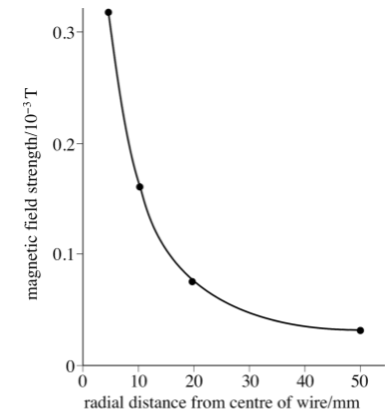Courses
Courses for Kids
Free study material
Offline Centres
MoreLast updated date: 03rd Dec 2023
Total views: 281.4k
Views today: 3.81k

# How does distance affect magnetic force ?Verified
281.4k+ views
Hint:Coulomb's Inverse Square Law of Magnetic Force, also known as Coulomb's Law of Magnetic Force, was introduced in $1785$ by Charles-Augustin de Coulomb, a French physicist and military engineer. For two isolated point poles, he interpreted the quantitative expression of power.

The force of attraction or repulsion between two magnetic poles of strengths ${m_1}$ and ${m_2}$ held at a distance r apart is directly proportional to the product of their pole strengths and inversely proportional to the square of the distance between them.
$F \propto \dfrac{{{m_1}{m_2}}}{{{r^2}}}$ or $F = \dfrac{{{\mu _o}}}{{4\pi }}\dfrac{{{m_1}{m_2}}}{{{r^2}}}$
$F \propto \dfrac{1}{{{r^2}}}$
Where $r$ is the distance between the magnets.Note:A unit magnetic pole is known as a magnetic pole that experiences a force of $1/2r$ newtons when placed one metre away from a very long straight conductor carrying a current of one ampere.